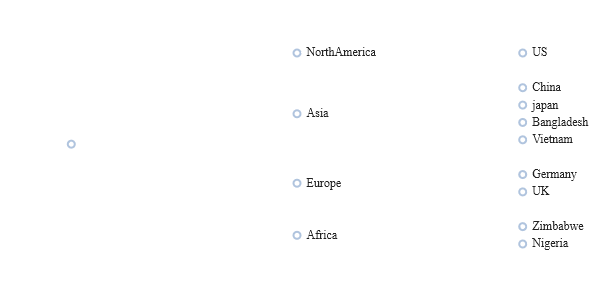Most chart formats are shown below to give a quick static image overview of the chart types.
The best place to see the charts is in the live help demo.

## Candlestick Chart

• The table should contain columns labelled open/high/low/close/volume.
• The table must atleast contain high/low or open/close to allow it to be drawn.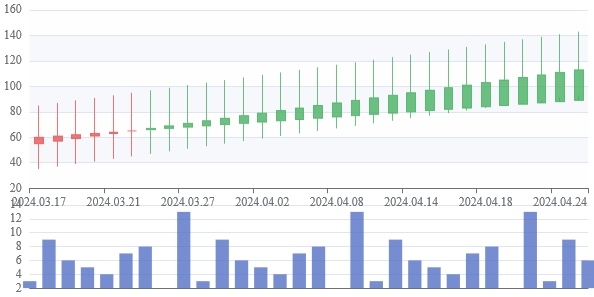## Area Chart

• The first string columns are used as category labels.
• Whatever numeric columns appear after the strings represents a separate series in the chart.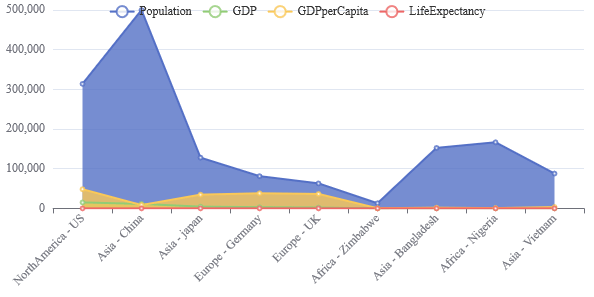## Line Chart

• The first string columns are used as category labels.
• Whatever numeric columns appear after the strings represents a separate series in the chart.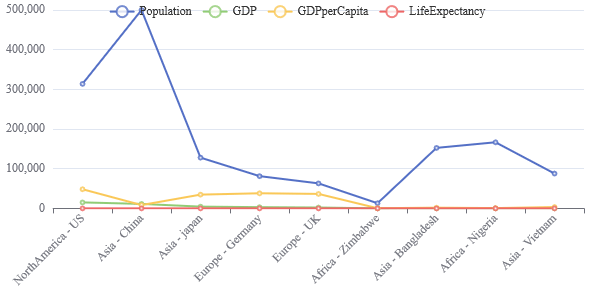## Bar Chart

• The first string columns are used as category labels.
• Whatever numeric columns appear after the strings represents a separate series in the chart.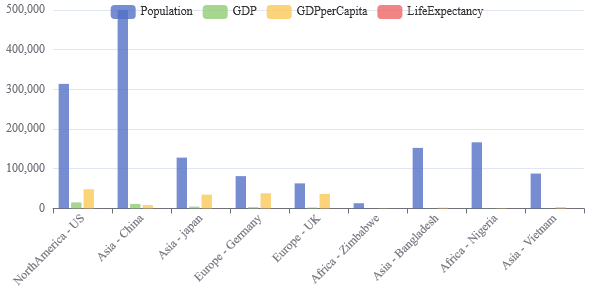## Horizontal Bar Chart

• The first string columns are used as category labels.
• Whatever numeric columns appear after the strings represents a separate series in the chart.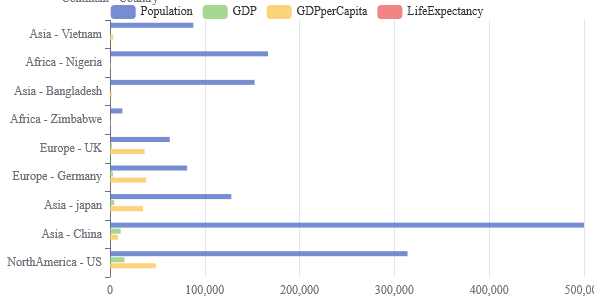## Stacked Bar Chart

• The first string columns are used as category labels.
• Whatever numeric columns appear after the strings represents a separate series in the chart.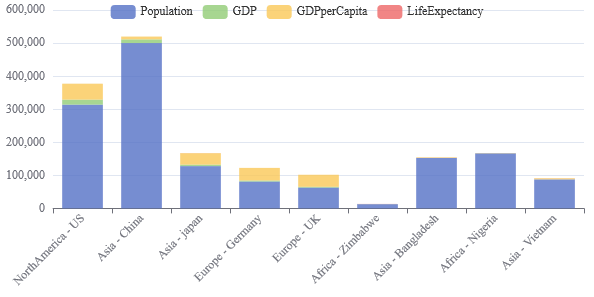## Horizontal Stacked Bar Chart

• The first string columns are used as category labels.
• Whatever numeric columns appear after the strings represents a separate series in the chart.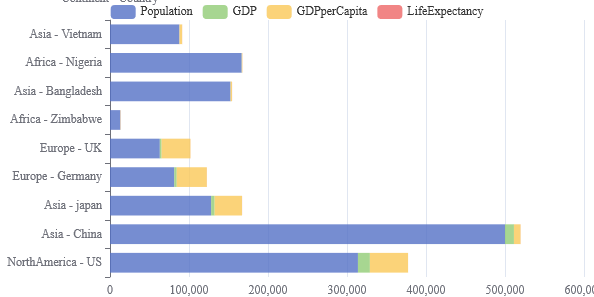## Pie Chart

• Each numeric column represents one pie chart. The title of each pie chart will be the column title.
• The segments of the pie chart use the string columns as a title where possible. If there are no string columns, row numbers are used.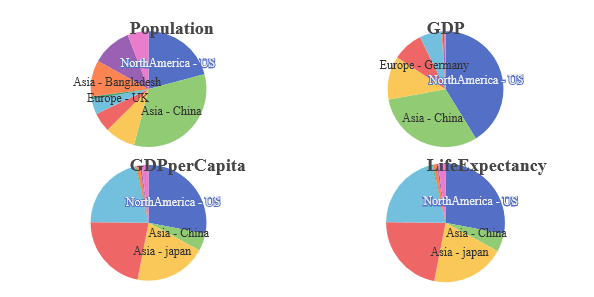## Scatter Graph

• Each numeric column represents one pie chart. The title of each pie chart will be the column title.
• The segments of the pie chart use the string columns as a title where possible. If there are no string columns, row numbers are used.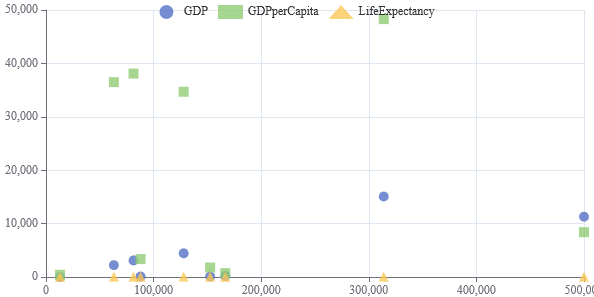• A radar chart requires 3 or more numeric columns to render sensibly.
• Each numeric column represents one spoke in the radar. The column titles are used as spoke titles.
• Each row in the data represents one circle withing the radar.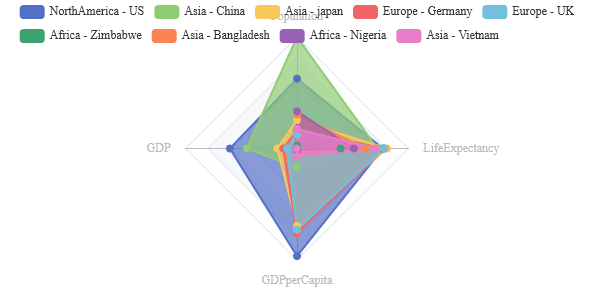## Calendar Heatmap

• The table should contain a date and atleast one numeric column.
• The first numeric column will be used as the value for that date.
• Dates should not be repeated. If they are the value selected is not guaranteed.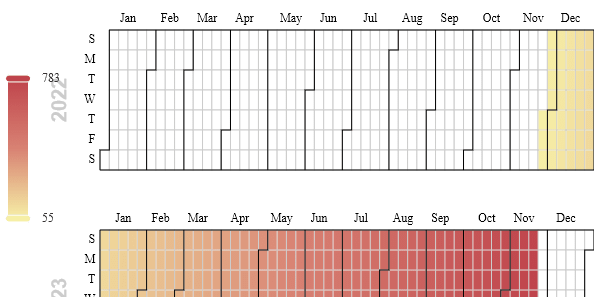## Heatmap

• Each numerical column in the table becomes one column in the chart.
• The numerical values represent the shading within the chart.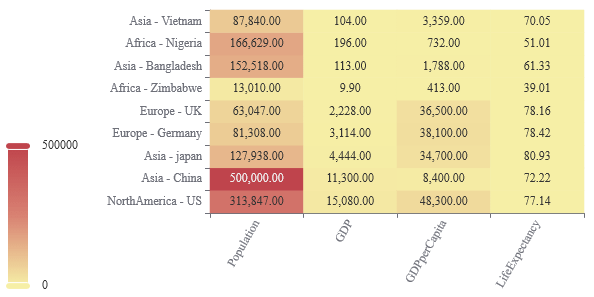## Treemap

• Starting from the left each string column is taken as one nesting level
• The first numerical column will be taken as size.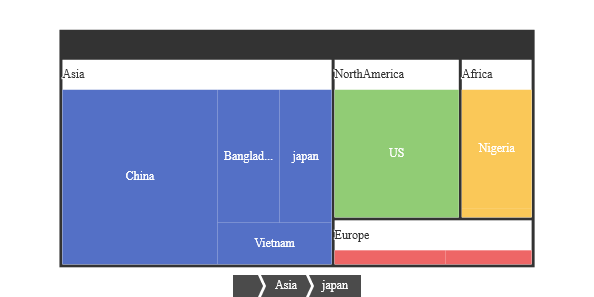## Boxplot

• Each numerical column in the table becomes one boxplot item in the chart.
• The min/max/median/Q1/Q3 are calculated from the raw data.
• This is inefficient as a lot more data is being passed than needed but useful for toggling an existing data set view quickly.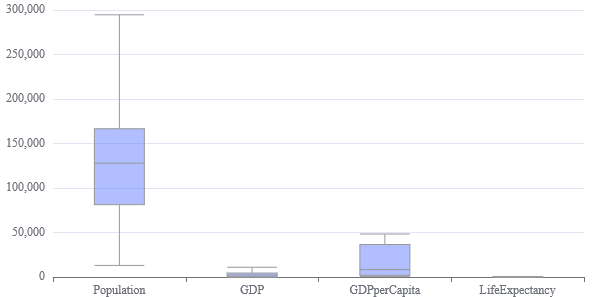## Sunburst

• Starting from the left each string column is taken as one nesting level
• The first numerical column will be taken as size.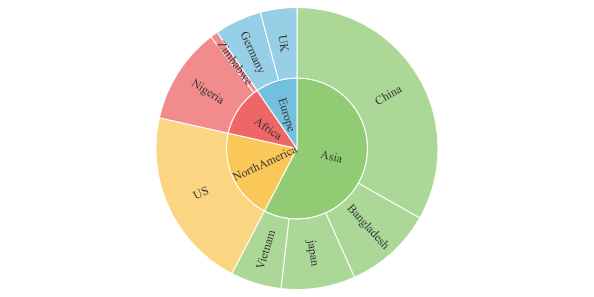## Tree

• Starting from the left each string column is taken as one nesting level
• The first numerical column will be taken as size.